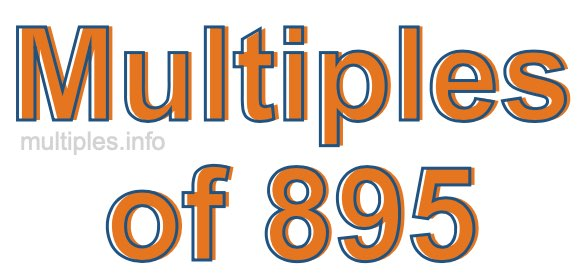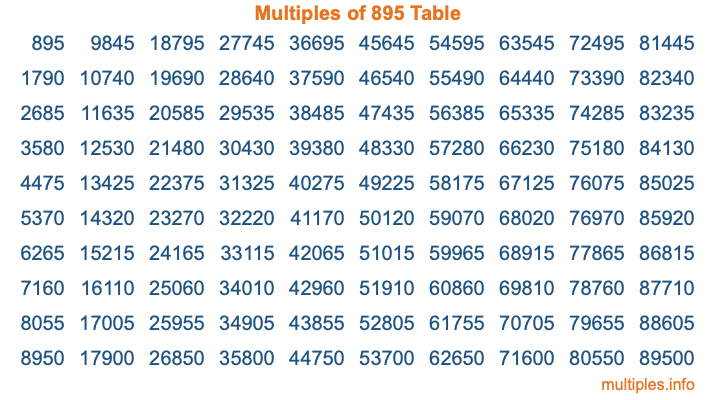Multiples of 895Welcome to the Multiples of 895 page. Here we will first teach you everything you will ever need to know about the multiples of 895, and then give you a study guide summary of everything we taught you to make sure you remember it all. Use this page to look up facts and learn information about the multiples of 895. This page will make you a multiples of eight hundred ninety-five expert!

Definition of Multiples of 895
Multiples of 895 are all the numbers that when divided by 895 equal an integer. Each of the multiples of 895 are called a multiple. A multiple of 895 is created by multiplying 895 by an integer.

Therefore, to create a list of multiples of 895, you start with 1 multiplied by 895, then 2 multiplied by 895, then 3 multiplied by 895, and so on for as long as you want. Thus, the list of the first five multiples of 895 is 895, 1790, 2685, 3580, and 4475. To see a larger list of multiples of 895, see the printable image of Multiples of 895 further down on this page. We also have a category where you can choose any nth multiple of 895.

Multiples of 895 Checker
The Multiples of 895 Checker below checks to see if any number of your choice is a multiple of 895. In other words, it checks to see if there is any number (integer) that when multiplied by 895 will equal your number. To do that, we divide your number by 895. If the the quotient is an integer, then your number is a multiple of 895.

Is  a multiple of 895?

Least Common Multiple of 895 and ...
A Least Common Multiple (LCM) is the lowest multiple that two or more numbers have in common. This is also called the smallest common multiple or lowest common multiple and is useful to know when you are adding our subtracting fractions. Enter one or more numbers below (895 is already entered) to find the LCM.

Check out our LCM Calculator if you need more details about the Least Common Multiple or if you need the LCM for different numbers for adding and subtraction fractions.

nth Multiple of 895
As we stated above, 895 is the first multiple of 895, 1790 is the second multiple of 895, 2685 is the third multiple of 895, and so on. Enter a number below to find the nth multiple of 895.

th multiple of 895

Multiples of 895 vs Factors of 895
895 is a multiple of 895 and a factor of 895, but that is where the similarities end. All postive multiples of 895 are 895 or greater than 895. All positive factors of 895 are 895 or less than 895.

Below is the beginning list of multiples of 895 and the factors of 895 so you can compare:

Multiples of 895: 895, 1790, 2685, 3580, 4475, etc.

Factors of 895: 1, 5, 179, 895

As you can see, the multiples of 895 are all the numbers that you can divide by 895 to get a whole number. The factors of 895, on the other hand, are all the whole numbers that you can multiply by another whole number to get 895.

It's also interesting to note that if a number (x) is a factor of 895, then 895 will also be a multiple of that number (x).

Multiples of 895 vs Divisors of 895
The divisors of 895 are all the integers that 895 can be divided by evenly. Below is a list of the divisors of 895.

Divisors of 895: 1, 5, 179, 895

The interesting thing to note here is that if you take any multiple of 895 and divide it by a divisor of 895, you will see that the quotient is an integer.

Multiples of 895 Table
Below is an image of the first 100 multiples of 895 in a table. The table is in chronological order, column by column. The first column has the first ten multiples of 895, the second column has the next ten multiples of 895, and so on.The Multiples of 895 Table is also referred to as the 895 Times Table or Times Table of 895. You are welcome to print out our table for your studies.

Negative Multiples of 895
Although not often discussed or needed in math, it is worth mentioning that you can make a list of negative multiples of 895 by multiplying 895 by -1, then by -2, then by -3, and so on, to get the following list of negative multiples of 895:

-895, -1790, -2685, -3580, -4475, etc.

Multiples of 895 Summary
Below is a summary of important Multiples of 895 facts that we have discussed on this page. To retain the knowledge on this page, we recommend that you read through the summary and explain to yourself or a study partner why they hold true.

There are an infinite number of multiples of 895.

A multiple of 895 divided by 895 will equal a whole number.

895 divided by a factor of 895 equals a divisor of 895.

The nth multiple of 895 is n times 895.

The largest factor of 895 is equal to the first positive multiple of 895.

895 is a multiple of every factor of 895.

895 is a multiple of 895.

A multiple of 895 divided by a divisor of 895 equals an integer.

895 divided by a divisor of 895 equals a factor of 895.

Any integer times 895 will equal a multiple of 895.

Multiples of a Number
Here you can get the multiples of another number, all with the same attention to detail as we did for multiples of 895 on this page.

Multiples of
Multiples of 896
Did you find our page about multiples of eight hundred ninety-five educational? Do you want more knowledge? Check out the multiples of the next number on our list!